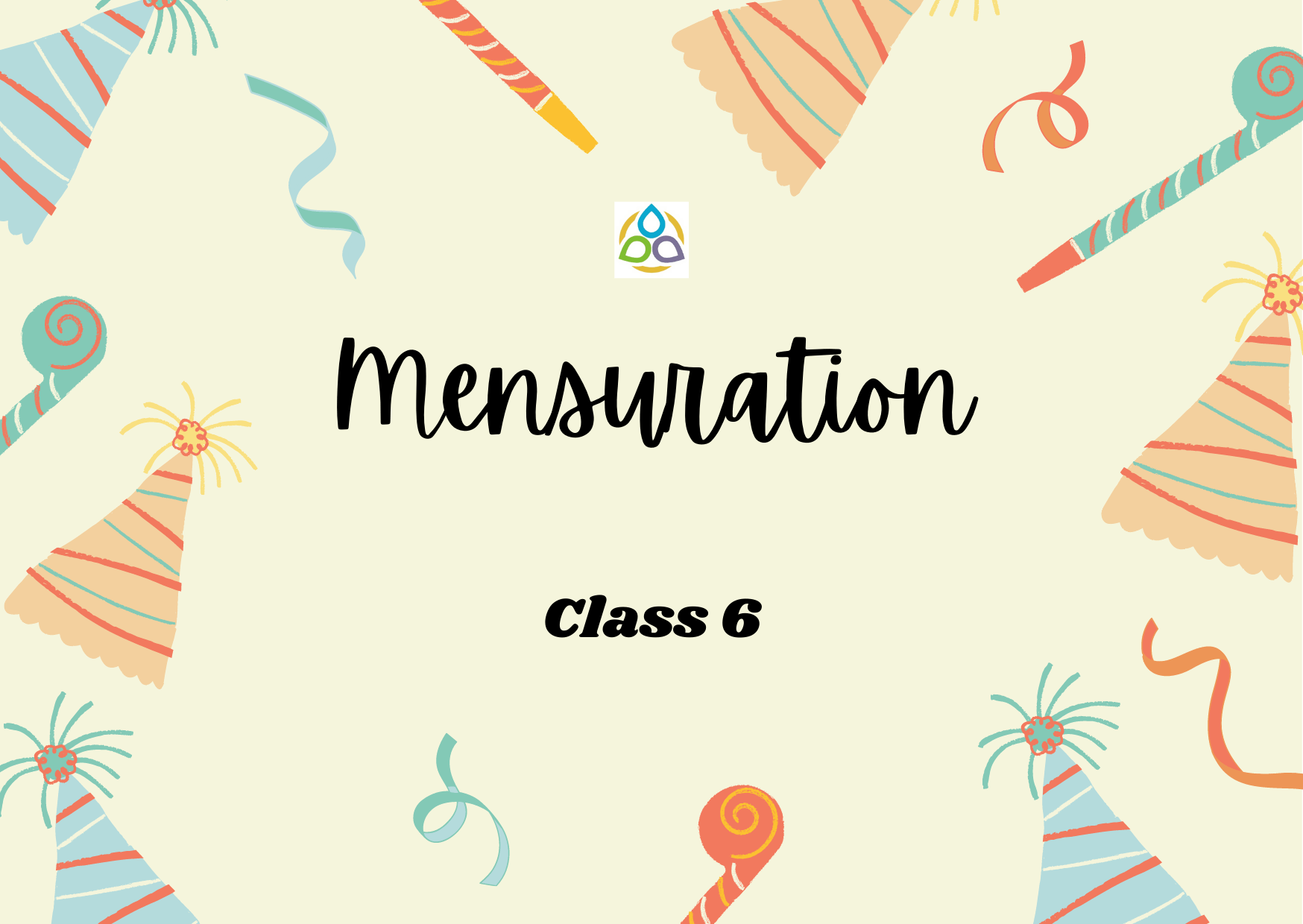# Mensuration

30. The distance covered in one round along the boundary of a closed figure is called

• (A) Length
• (C) Perimeter
• (D) Area

29. The amount of region enclosed by a plane closed figure is called its

• (A) Diagonal
• (C) Perimeter
• (D) Area

28. To find the cost of painting a wall we need to find the perimeter of the wall.

• (A) Length
• (C) Perimeter
• (D) Area

27. To find the cost of a frame of a picture, we need to find the perimeter of the picture.

• (A) Diagonal
• (C) Perimeter
• (D) Area

26. An engineer who plans to build a compound wall on all sides of a house must find the area of the compound.

• (A) Length
• (C) Perimeter
• (D) Area

25. A farmer who wants to fence his field, must find the perimeter of the field.

• (A) Length
• (B) Diagonal
• (C) Perimeter
• (D) Area

24. Area of a rectangle with length 5 cm and breadth 3 cm is

• (A) 14 sq cm
• (B) 15 sq cm
• (C) 16 sq cm
• (D) 20 sq cm

23. Perimeter of a triangle with sides 4.5 cm, 6.02 cm and 5.38 cm is

• (A) 15.02 cm
• (B) 15.9 cm
• (C) 15.09 cm
• (D) 15.01 cm

22. Perimeter of a regular octagon of side 6 cm

• (A) 12 cm
• (B) 48 cm
• (C) 24 cm
• (D) 64 cm

21. The perimeter of a regular pentagon is 1540 cm. How long is its each side?

• (A) 307 cm
• (B) 308 cm
• (C) 310 cm
• (D) 364 cm